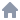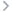Top Techniques to Ace the SAT Math Section: Your Comprehensive Guide# Top Techniques to Ace the SAT Math Section: Your Comprehensive Guide

Jun 24, 2023

Preparing for the SAT Math section might feel intimidating, but with strategic studying, a firm grasp of mathematical concepts, and the right tools, you can confidently tackle the test and aim for a top score. Here's your ultimate guide to mastering the SAT Math section.

## 1. Understand the SAT Math Test Structure

Knowing the structure of the SAT Math section is the foundation of your study plan. This section is divided into two parts: Math Test - Calculator and Math Test - No Calculator. It covers topics such as algebra, problem-solving, data analysis, and some areas of advanced math.

## 2. Strengthen Your Core Math Skills

Revise and solidify your knowledge of fundamental math concepts. Focus on areas such as linear equations, fractions, percentages, ratios, and geometry. Apps like Anki and Quizlet are excellent resources for creating flashcards for formulas and concepts.

## 3. Get Familiar with Word Problems

The SAT Math section includes word problems that require you to apply mathematical principles to real-world situations. Practice solving word problems to get comfortable with translating textual information into mathematical terms.

## 4. Practice with Real SAT Math Questions

Utilize real SAT Math questions for practice. The College Board offers sample questions and practice tests that provide an authentic test-taking experience. Review your answers and understand your mistakes to avoid them in the future.

## 5. Learn to Use Your Calculator Efficiently

Know when to use your calculator and when to solve problems manually. Familiarize yourself with your calculator’s functions and shortcuts to save time during the test.

Automating flashcards creation for math formulas and concepts can save you time and ensure you don’t miss important information. Consider using a tool like Paperclips, which can generate flashcards from any web page or uploaded PDF.

## 7. Manage Your Time Effectively

Time management is key in the SAT Math section. Practice under timed conditions to get a feel for the pace you need to maintain during the actual test.

With these strategies and resources, you're well-equipped to conquer the SAT Math section. Remember, successful test-taking is a blend of knowledge, strategy, and practice. Good luck with your SAT preparation!

Paperclips Copilot is the smartest way to create flashcards on the web.Top Techniques to Ace the SAT Math Section: Your Comprehensive Guide# Top Techniques to Ace the SAT Math Section: Your Comprehensive Guide

Jun 24, 2023

Preparing for the SAT Math section might feel intimidating, but with strategic studying, a firm grasp of mathematical concepts, and the right tools, you can confidently tackle the test and aim for a top score. Here's your ultimate guide to mastering the SAT Math section.

## 1. Understand the SAT Math Test Structure

Knowing the structure of the SAT Math section is the foundation of your study plan. This section is divided into two parts: Math Test - Calculator and Math Test - No Calculator. It covers topics such as algebra, problem-solving, data analysis, and some areas of advanced math.

## 2. Strengthen Your Core Math Skills

Revise and solidify your knowledge of fundamental math concepts. Focus on areas such as linear equations, fractions, percentages, ratios, and geometry. Apps like Anki and Quizlet are excellent resources for creating flashcards for formulas and concepts.

## 3. Get Familiar with Word Problems

The SAT Math section includes word problems that require you to apply mathematical principles to real-world situations. Practice solving word problems to get comfortable with translating textual information into mathematical terms.

## 4. Practice with Real SAT Math Questions

Utilize real SAT Math questions for practice. The College Board offers sample questions and practice tests that provide an authentic test-taking experience. Review your answers and understand your mistakes to avoid them in the future.

## 5. Learn to Use Your Calculator Efficiently

Know when to use your calculator and when to solve problems manually. Familiarize yourself with your calculator’s functions and shortcuts to save time during the test.

Automating flashcards creation for math formulas and concepts can save you time and ensure you don’t miss important information. Consider using a tool like Paperclips, which can generate flashcards from any web page or uploaded PDF.

## 7. Manage Your Time Effectively

Time management is key in the SAT Math section. Practice under timed conditions to get a feel for the pace you need to maintain during the actual test.

With these strategies and resources, you're well-equipped to conquer the SAT Math section. Remember, successful test-taking is a blend of knowledge, strategy, and practice. Good luck with your SAT preparation!

Paperclips Copilot is the smartest way to create flashcards on the web.Top Techniques to Ace the SAT Math Section: Your Comprehensive Guide# Top Techniques to Ace the SAT Math Section: Your Comprehensive Guide

Jun 24, 2023

Preparing for the SAT Math section might feel intimidating, but with strategic studying, a firm grasp of mathematical concepts, and the right tools, you can confidently tackle the test and aim for a top score. Here's your ultimate guide to mastering the SAT Math section.

## 1. Understand the SAT Math Test Structure

Knowing the structure of the SAT Math section is the foundation of your study plan. This section is divided into two parts: Math Test - Calculator and Math Test - No Calculator. It covers topics such as algebra, problem-solving, data analysis, and some areas of advanced math.

## 2. Strengthen Your Core Math Skills

Revise and solidify your knowledge of fundamental math concepts. Focus on areas such as linear equations, fractions, percentages, ratios, and geometry. Apps like Anki and Quizlet are excellent resources for creating flashcards for formulas and concepts.

## 3. Get Familiar with Word Problems

The SAT Math section includes word problems that require you to apply mathematical principles to real-world situations. Practice solving word problems to get comfortable with translating textual information into mathematical terms.

## 4. Practice with Real SAT Math Questions

Utilize real SAT Math questions for practice. The College Board offers sample questions and practice tests that provide an authentic test-taking experience. Review your answers and understand your mistakes to avoid them in the future.

## 5. Learn to Use Your Calculator Efficiently

Know when to use your calculator and when to solve problems manually. Familiarize yourself with your calculator’s functions and shortcuts to save time during the test.

Automating flashcards creation for math formulas and concepts can save you time and ensure you don’t miss important information. Consider using a tool like Paperclips, which can generate flashcards from any web page or uploaded PDF.

## 7. Manage Your Time Effectively

Time management is key in the SAT Math section. Practice under timed conditions to get a feel for the pace you need to maintain during the actual test.

With these strategies and resources, you're well-equipped to conquer the SAT Math section. Remember, successful test-taking is a blend of knowledge, strategy, and practice. Good luck with your SAT preparation!